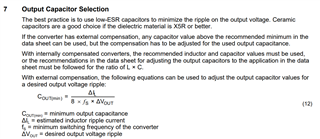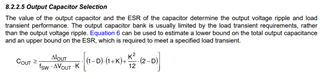If you have a related question, please click the "Ask a related question" button in the top right corner. The newly created question will be automatically linked to this question.

# LMR33630-Q1: the different of the calculation of Cout value.

Part Number: LMR33630-Q1
Other Parts Discussed in Thread: LMR33630

Hi team,

My customer E-LEAD is asking the question that they notice if follow TI SLVA477B to calculate Cout(min). the formula will be:If follow the datasheet of LMR33630-Q1. the formula will be:I would like to know what different between two formula. As I know that the first one is calculated by roughly Cout's ΔQ = C x ΔV = ΔI x T = 1/2 x T/2 x ΔiL/2 to get the answer C = ΔiL / (8 x fs x ΔVo). but I can't understand the second one how to get the K ripple factor. Would you please help to explain it? thanks.

BR,

Jimmy

• Hello

The equations from either data sheet are only very rough approximations to the required output capacitance.

Neither equation can be valid for all operating conditions. They are included in the data sheet to give a starting

point to provide a stable regulator.

Regarding the equation in the LMR33630 data sheet, it is based on an inductance limited response.

For the LMR33630 we have a calculator tool on the product page that should be used to determine the

minimum output capacitance.  The tool can also be used to determine the loop phase and gain margin

based on a desired output capacitance.  The tool is much more accurate and flexible than the equation(s) in the

data sheet.

Alternatively, the output capacitance in the data sheet table can be used as a starting point.

Thanks

• Hi Frank,

I would like know the detail of the second formula. since my customer want to know how did this formula come about.

BR,

Jimmy

• Hello

See attached

Thanks

05229206.pdf

• Hi Frank,

thanks a lots!

BR,

Jimmy